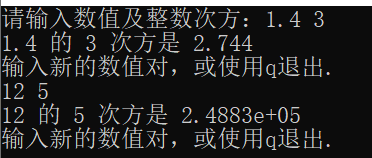2022
07-02

# 用c语言编写一个幂函数(c语言实现幂函数)for(i = 1; i<=p;i++)

pow *= n;

1.函数的参数是什么

2.函数的返回值是什么

double power(double n, int p);

```#include<stdio.h> double power(double n, int p); int main(void){ double x, xpow; int exp; printf("请输入数值及整数次方："); while(scanf("%lf %d",&x,&exp) == 2){
xpow = power(x,exp); printf("%.3g 的 %d 次方是 %.5g\n",x,exp,xpow); printf("输入新的数值对，或使用q退出.\n");
}
} double power(double n, int p){ double pow = 1; int i; for(i = 1;i<=p;i++){ pow *= n;
} return pow;
}```